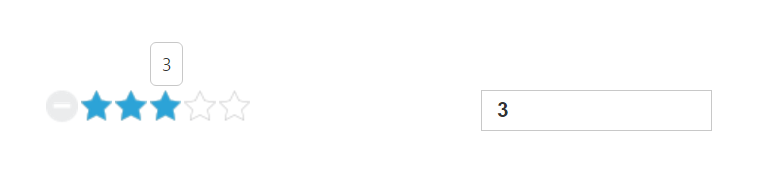# Data-binding

28 Jun 20172 minutes to read

In data binding concept of Rating control, need to set the value property using two way binding that is you need to assign the rating value using scope variable.

The following example depicts the way to bind data to the Rating control.

Add the following code to render Rating with data binding.

• HTML
• ``````<body ng-controller="RatingCtrl">
<table>
<th>
<div id="control">
<input id="apiRating" type="text" class="rating" ej-rating e-value="ratingValue">
</div>
</th>
<th>
<div id="binding">
<input type="text" class="input ejinputtext" name="rating" value="" ng-model="ratingValue" />
</div>
</th>
</table>
</body>``````
• JAVASCRIPT
• ``````angular.module('ratingApp', ['ejangular'])
.controller('RatingCtrl', function (\$scope) {
\$scope.ratingValue = 3;
});``````
• CSS
• ``````<style type="text/css" class="cssStyles">
#binding {
margin-left: 150px;
}
.control {
margin-top: 10px;
}
.input {
height: 27px;
text-indent: 10px;
width: 81%;
}
</style>``````

The following screenshot displays the output of Rating with data binding support.